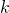# Modified inertial Mann’s algorithm and inertial hybrid algorithm for k-strict pseudo-contractive mappings

### Full PDFcarpathian_2023_39_1_27_43

In this work, we introduce and study the modified inertial Mann’s algorithm and inertial hybrid algorithm for approximating some fixed points of a-strict pseudo-contractive mapping in Hilbert spaces. Weak convergence to a solution of fixed-point problems for a-strict pseudo-contractive mapping is obtained by using the modified inertial Mann’s algorithm. In order to obtain strong convergence, we introduce an inertial hybrid algorithm by using the inertial extrapolation method mixed with the convex combination of three iterated vectors and forcing for strong convergence by the hybrid projection method for a-strict pseudo-contractive mapping in Hilbert spaces. The strong convergence theorem of the proposed method is proved under mild assumptions on the scalars. For illustrating the performance of the proposed algorithms, we provide some new nonlinear-strict pseudo-contractive mappings which are not nonexpansive to create some numerical experiments to show the advantage of the two new inertial algorithms for a-strict pseudo-contractive mapping.